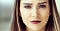Source: https://us.norton.com/internetsecurity-iot-how-facial-recognition-software-works.html

Last couple months, I had an opportunity to enroll in Udacity’s Deep Learning NanoDegree program. Thanks for Facebook PyTorch Scholarship. The program is ending but not the learning. The program encourage participants to keep learning, practicing and sharing. Writing is one good way to do so. I will publish a series about deep learning applications using PyTorch. Hope it be benefit to anyone as well as me. 😃

In this article, we will see how to create models such as Multi-layer Perceptron (MLP) and Convolutional Neural Network (CNN) ) to detect facial keypoints and how well they perform, how to do image augmentations, how to create data loading and processing, and how to train and deploy model using PyTorch.

Codes and more details are here. The notebook can be ran on CoLab. Any comments and suggestions are very welcome and appreciated. All credentials go to below references.

Thai version (ภาษาไทย) coming soon!

# Facial Keypoints Detection

Detecting key positions on face image is useful in several applications such as tracking face in image or video, analyzing facial expression, face recognition, and so on. In this article, we will use data provided by Kaggle’s Facial Keypoints Detection competition and evaluate our predictions through it.

# Explore Data

There’re two data files-`train.csv` for training `test.csv` and for testing. Let’s see what’s inside.

## Training Data

There’re 7,094 images in training data. The last field, Image, consists of pixels as integers (0–255) separated by space. The images are 96 x 96 pixels. The first 30 fields are labels, the coordinates (x, y) of 15 keypoints:

• Eyes: left_eye_center, right_eye_center, left_eye_inner_corner, left_eye_outer_corner, right_eye_inner_corner, right_eye_outer_corner
• Eyebrows: left_eyebrow_inner, left_eyebrow_outer, right_eyebrow_inner, right_eyebrow_outer
• Nose and Mouth: nose_tip, mouth_left_corner, mouth_right_corner, mouth_center_top, mouth_center_bottom.
`import pandas as pdfrom pathlib import Pathdata_dir = Path('./data')train_data = pd.read_csv(data_dir/'training.csv')train_data.T`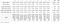Fig. 1 Training Data (Transposed)

From Fig 1, we can see there’re some missing-keypoints data (NaN) in our training data. We will check it later.

We create helper functions, `show_keypoints()` to show keyponts on image and `show_images()` to display images from pandas dataframes with or without keypoints.

Let’s see how the images and keypoints look like. The keypoints are marked by red-dots. Fig 2 shows samples having all 15 keypoints.

`show_images(train_data, range(4))`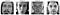Fig. 2: Training Data Samples

Let’s randomly see how missing-keypoint samples look like.

`missing_any_data = train_data[train_data.isnull().any(axis=1)]idxs = np.random.choice(missing_any_data.index, 4)show_images(train_data, idxs)`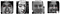Fig 3: Missing-Keypoints Samples

Fig 3 shows some missing-keypoints samples. As you can see, besides missing-keypoint, there are blur (#6319), cropped (#1546), and even missed-annotated sample (#2199). If we want to use these samples, we need to decide how to handle missing data and take into account of these diverse-quality samples.

Test Data

For test data, there’re 1,783 images with only two field-ImageId and Image.

`test_data = pd.read_csv(data_dir / 'test.csv')test_data.head()`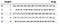Fig. 4: Test Data

# Base Case: Drop Any-Missing-Keypoints Samples

We will begin with the samples having all 15 keypoints as base case. There’re 2,140 samples having all keypoints in training data. We will use this dataset as our base case.

`train_df = train_data.dropna()train_df.info()`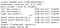Fig. 5: Base Case Training Data

# Preprocessing Data

One important process in data science pipeline is data preprocessing. PyTorch provides `Dataset` and `DataLoader` classes to make it easy and, hopefully, to make your code more readable.

## `Dataset` and `DataLoader`

`Dataset` allows you incorporate data preprocessing process through callable classes and `DataLoader` makes it easy to manage how data be feed into model more conveniently and efficiently.

## Create FaceKeypointsDataset

We create `FaceKeypointsDataset` as a subclass of` torch.utils.data.Dataset` and override `__len__` method to support` len(dataset)` and` __getitem__` method to support `dataset[i]` for data iteration which’s not stored all data in memory at once but read as required.

Sample of our dataset will be a `dict` `{'image': image, 'keypoints': keypoints}`. Our dataset will take an optional argument `transform` so that any required processing can be applied on the sample.

## Transforms

Before feeding into the model, numpy array images need to be normalized and converted to `Tensor`. We will create `transform` as callable classes named `Normalize` and `ToTensor`.

## Split training data into train and validation sets

We will write a helper function to prepare train loader and validation loader from train dataset.

Now we’re ready to create `Dataset` and `DataLoader` for training, validation and test as well. We also compose `Normalize` and `ToTensor` transforms by using `torchvision.transforms.Compose`

# MLP Model

For base case, let’s begin with Multi-Layer Perceptrons (MLP). In PyTorch, we can construct neural network model by subclass `nn.Module` and define `__init__` and `forward` methods. Our MLP will have couple hidden layers and one output layer. Each hidden layer will consist of fully-connected layer with activation function and dropout layer.

Our model will have input size 9,216 (96 * 96) and two fully-connected layers, 128 and 64 units each, with ReLu activation and dropout with probability 0.1. The output size is 30 which is the number of total keypoints in x and y.

# Train the Network

In PyTorch, we can specify if the network will be trained on GPU or CPU by defining the device and set the model to it.

`device =torch.device(‘cuda’ if torch.cuda.is_available() else ‘cpu’)model = MLP(input_size=IMG_SIZE*IMG_SIZE, output_size=30,             hidden_layers=[128, 64], drop_p=0.1)model = model.to(device)`

For the training, we need to specify objective loss function or criterion and optimizer. We will define Mean Square Errors (MSE) as criterion and Adam with learning rate (lr) equal 0.003 as optimizer.

`from torch import optimcriterion = nn.MSELoss()optimizer = optim.Adam(model.parameters(), lr=0.003)`

For convenience, we will wrap up the training and validation processes by creating `train` function which will train, validate, save the minimum-validation-RMSE model, and return the training and validation RMSEs by epoch.

Now, let’s train the base case for 50 epochs and save the model as “model.pt”

`train_losses, valid_losses = train(train_loader, valid_loader,                                   model,criterion, optimizer,                                   n_epochs=50,                                   saved_model='model.pt')`

# Predictions

We will provide two functions `predict` , to predict keypoints of images from specific model, and `view_pred_df`, to display keypoints from test dataframe.

Now, let’s view how our model predict keypoints on test set images.

`# Load the minimum validation loss modelmodel.load_state_dict(torch.load('model.pt'))predictions = predict(test_loader, model)columns = train_df.drop('Image', axis=1).columnsview_pred_df(columns, test_df, predictions)`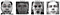Fig. 7: Predicted Keypoints on Test Data, MLP Model

With validation loss about 7.8 and what we see on images, it seems our predictions are not effective enough. Let’s see how would they score on Kaggle.

# Evaluation

To submit our predictions, we will use `create_submission` function to prepare the csv file as required by Kaggle for submission.

Now create `submission.csv` and submit to Kaggle at https://www.kaggle.com/c/facial-keypoints-detection/submit

`create_submission(predictions)`

The score is RMSE, same as our loss. It’s not so good, let’s see if we can improve it by increasing various samples with data augmentation .

# Augmentation

Data augmentation can help increase amount of relevant data for training. Besides preparing data as we did, we can compose data augmentations using `transform` object as well. For images, we can do many ways-flip, resize, crop, rotate, etc. Let’s try randomly flip image horizontally by create `RandomHorizontalFlip` transform.

All we need to do is just add `RandomHorizontalFlip` to `transforms.compose` and prepare `trainset`, `train_loader` and `valid_loader` as we did earlier.

Let’s run the training using same MLP model, criterion, and optimizer for 50 epochs and save it to `aug_model.pt`

`model = MLP(input_size=IMG_SIZE*IMG_SIZE, output_size=30,             hidden_layers=[128, 64], drop_p=0.1)model = model.to(device)criterion = nn.MSELoss()optimizer = optim.Adam(model.parameters(), lr=0.003)train(aug_train_loader, aug_valid_loader, model, criterion,       optimizer, n_epochs=50, saved_model='aug_model.pt')`
`model.load_state_dict(torch.load('aug_model.pt'))predictions = predict(test_loader, model)columns = train_df.drop('Image', axis=1).columnsview_pred_df(columns, test_df, predictions)`

The model gives the worsen loss. The visualization looks not different from base case. However, it seem do better on Kaggle test samples.

# Convolutional Neural Network (CNN)

Next, we will try CNN model which is more suitable for image problems. Our CNN will have three convolutional layers with ReLu activation and max-pooling layer each, followed by two 128-unit fully connected layers with dropout layer each. You can learn more about CNN here.

In PyTorch, we can construct CNN model by subclass `nn.Module` as well. The CNN class will take output-the number of keypoints-as argument.

Now let’s train CNN model with augmented data.

`model = CNN(outputs=30)model = model.to(device)criterion = nn.MSELoss()optimizer = optim.Adam(model.parameters(), lr=0.003)train(aug_train_loader, aug_valid_loader, model, criterion,  optimizer, n_epochs=50, saved_model='aug_cnn.pt')`
`model.load_state_dict(torch.load('aug_cnn.pt'))predictions = predict(test_loader, model)create_submission(predictions,                   pred_file='data/aug_cnn_preds.csv',                   sub_file='data/aug_cnn_submission.csv')view_pred_df(columns, test_df, predictions)`

The CNN model improve predictions quite well. Both visualization and Kaggle scores improve quite well.

# 2 Models-2 Datasets

So far we just use 2,140 images from total 7,094 images in original training data. What’s about the rest? If we look at our training data, we can see there’re two groups of keypoints-one with about 2,000 samples and one with about 7,000 samples. To make uses of them, we will build separate models based on samples of each groups.

`train_data.info()`

We will group samples into two groups, L (Large)-for the keypoints with about 7,000 samples and S (Small)-for the keypoints with about 2,000 samples. We will define this in datasets dictionary.

We need to modify our `RandomHorizontalFlip` to take `dataset` as an argument.

## L Model

Now, let’s select L dataset (7,000 samples), preprocess data, create model, define criterion and optimizer, train the model, and view predictions.

`# Select L dataL_aug_df = train_data[datasets[‘L’]].dropna()L_aug_df.info()`
`outputs = len(datasets['L']) - 1model = CNN(outputs)model = model.to(device)criterion = nn.MSELoss()optimizer = optim.Adam(model.parameters(), lr=0.003)train(L_aug_train_loader, L_aug_valid_loader, model, criterion, optimizer, n_epochs=50, saved_model='L_aug_cnn.pt')`
`model.load_state_dict(torch.load('L_aug_cnn.pt'))L_predictions = predict(test_loader, model)L_columns = L_aug_df.drop('Image', axis=1).columnsview_pred_df(L_columns, test_df, L_predictions)`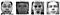Predictions for L Dataset

## S Model

S dataset have 2,155 non-missing samples.

`# Select S dataS_aug_df = train_data[datasets['S']].dropna()S_aug_df.info()`
`outputs = len(datasets['S']) - 1model = CNN(outputs)model = model.to(device)criterion = nn.MSELoss()optimizer = optim.Adam(model.parameters(), lr=0.003)train(S_aug_train_loader, S_aug_valid_loader, model, criterion, optimizer, n_epochs=50, saved_model='S_aug_cnn.pt')`
`model.load_state_dict(torch.load('S_aug_cnn.pt'))S_predictions = predict(test_loader, model)S_columns = S_aug_df.drop('Image', axis=1).columnsview_pred_df(S_columns, test_df, S_predictions)`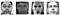Prdictions for S Dataset

## Combine L & S model predictions

Now, we combine both predictions and submit.

`predictions = np.hstack((L_predictions, S_predictions))columns = list(L_columns) + list(S_columns)view_pred_df(columns, test_df, predictions)`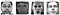Combined L and S Predictions
`create_submission(predictions, columns=columns,                   pred_file='data/2models_preds.csv',                   sub_file='data/2models_submission.csv')`

Wow!, the approach gives us a better result.

# Conclusions

That’s what I would like to share about PyTorch. We have learned to :

• preprocess data and create `transforms` by using `Dataset` and `DataLoader.`
• construct models-MLP and CNN by using `nn.Module`
• define criterion and optimizer, and train models.
• save and load models.
• evaluate and deploy models.

What’s next we can do?

There’re still plenty rooms to improve our predictions. We may consider:

• Handling missing values and poor-annotated samples.
• More augmentations-rotate, blur, crop, resize, brightness, contrast, etc.
• Hyperparameters tuning-number layers, epochs, learning rate, etc.
• Different model architects and transfer learning.
• More sub-datasets or ensembles.

Thanks for reading. Any comments and suggestions are welcome. And don’t forget we can learn better together.

If you find this article helpful, kindly give it a clap . 👏

# Resources and references:

Written by

## Diving in Deep

#### The journey from machine learning 101 to AlphaGO deep learning

Welcome to a place where words matter. On Medium, smart voices and original ideas take center stage - with no ads in sight. Watch
Follow all the topics you care about, and we’ll deliver the best stories for you to your homepage and inbox. Explore
Get unlimited access to the best stories on Medium — and support writers while you’re at it. Just \$5/month. Upgrade